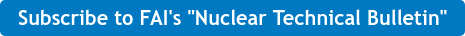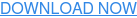### nuclear### chemical### Combustible dust### testing### news#### Recent Posts

Fauske & Associates, LLC (FAI) recently performed a cable aging project which required energizing a medium voltage power cable at frequencies beyond the conventional 50/60 Hz. The purpose of the project was to observe aging effects while the cable was energized underwater, at elevated temperatures and frequencies.

One of the challenges was deciding the method to energize the circuit. The cable’s capacitive reactance decreases as the applied frequency increases, resulting in higher reactive current demands from a power supply which limits the selection of power supplies.
A technique used for medium to high voltage cable testing applications is to utilize a parallel resonant test system. A parallel resonant circuit is an inductor and a capacitor in parallel driven by a voltage supply at a frequency where the inductor and capacitor reactances cancel each other because they are 180° out of phase and equal in magnitude. The frequency at which this occurs is known as the resonant frequency. The following equations demonstrate the relationship between inductive reactance (XL), capacitive reactance (XC) and resonant frequency:Equation 1
Where:
L is the inductance value
C is the capacitance value
f is the resonant frequency

Equation 1 ultimately yields the resonant frequency equation:Equation 2

A coiled cable was used as the inductor and the capacitor was that of the cable under test. For this test configuration a transformer was needed to elevate the voltage to the required test value.

When in resonance the circuit current is due to the resistance only. The inductor and capacitor exchange energy every half cycle and therefore the current follows the same pattern. No reactive current is supplied to the inductor and capacitor which was the objective of the circuit.

The following photographs show the example parallel resonant test system used at FAI. Figure 1 shows a pole top transformer capable of stepping up the low input voltage to medium and high voltage levels and handling the reactive power levels of the circuit. To the right of the transformer in Figure 1 is an inductor coil. The gauge of the inductor coil must be properly sized to carry the reactive current values of the circuit. Figure 2 below is an example medium voltage power cable used for testing.Figure 1                                                                                                                  Figure 2

In summary, the circuit characteristics of a parallel resonant circuit at its resonant frequency allow testing of medium voltage cables at elevated frequencies while requiring relatively low input reactive power.

#cable health, #nuclear #cable and wire## Is My Dust Combustible?